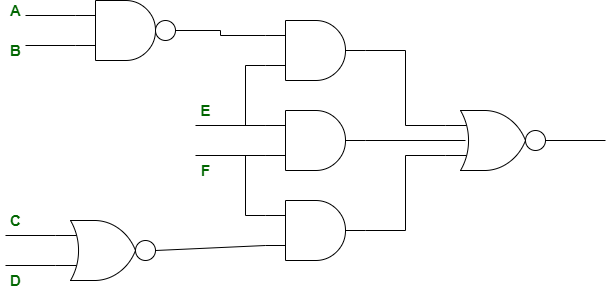GFG App
Open AppBrowser
Continue

# ISRO | ISRO CS 2020 | Question 52

Consider the following circuitThe function by the network above is
(A) (AB)’E + EF + (CD)’F
(B) (E’ + ABF’)(C + D + F’)
(C) ((AB)’ + E)(E’ + F’)(C + D + F’)
(D) (A + B)E’ + (EF)’ + CDF’

Explanation: The function by the network above is,

```= [(AB)'E + EF + (C+D)'.F]'
= [(AB)'E]'.[EF]'.[(C+D)'.F]'
= [(AB)+E'].[E'+F'].[C+D+F']
= (E' + ABF')(C + D + F')```

Option (B) is correct.

Quiz of this Question

My Personal Notes arrow_drop_up